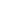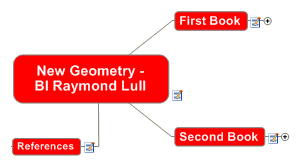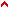New Geometry - Bl Raymond LullGod, You are the supreme truth, wisdom and love. With your virtue and in your honor, we now begin this new and concise Geometry.
As it is lovable to discover things quickly, let us now briefly investigate the secrets and natural truths of sensible and imaginable measurements, and let us carry out this investigation following the process of the General Art. In this art, we want to use common, easy to understand terms, so that those who do not know the terms of ancient Geometry can understand this science, and so we also deal with plain numbers without entering into algorithmic formulas.
HOW THIS SCIENCE IS DIVIDED
This science is divided into two books.
The first book has three parts:
1. The first part deals with the squaring of the circle and the triangulature of the square.
2. The second part is about extending the lines of the circle, the square and the triangle.
3. The third part is about multiplying figures.

The second book has three parts:
1.
In the first part, we describe the usefulness of this science.
2. The second is about the principles of Geometry and the conclusions drawn from its principles.
3. The third part deals with questions and solutions of some doubtful issues of Geometry.Top